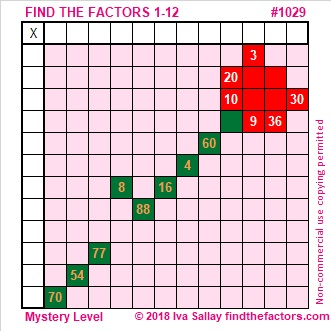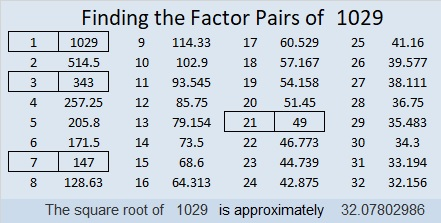# 1029 A Rose for Your Valentine

A dozen roses can be pretty pricey around Valentine’s Day, but at least one website asserts that a single rose can make just as big a statement and just as big an impact. Today’s mystery level puzzle looks like a single rose. I hope you will enjoy its beauty even if its thorns are prickly.Print the puzzles or type the solution in this excel file: 12 factors 1028-1034

Here are a few facts about the number 1029:

It’s easy to see that 1029 can be evenly divided by 3 because 1 + 0 + 2 + 9 = 12, a number divisible by 3.

It’s not quite as easy to tell that it can be evenly divided by 7:
It is because 102 – 2(9) = 102 – 18 = 84, a number divisible by 7.

I like the way 1029 looks when it is written in some other bases:
It’s 4433 in BASE 6 because 4(6³) + 4(6²) + 3(6¹) + 3(6⁰) = 4(216 + 36) + 3(6 + 1) = 1029,
3000 in BASE 7 because 3(7³) = 3(343) = 1029,
399 in BASE 17 because 3(17²) + 9(17) + 9(1) = 3(289 + 51 + 3) = 3(343) = 1029
333 in BASE 18 because 3(18² + 18 + 1) = 3(343) = 1029

• 1029 is a composite number.
• Prime factorization: 1029 = 3 × 7 × 7 × 7, which can be written 1029 = 3 × 7³
• The exponents in the prime factorization are 1 and 3. Adding one to each and multiplying we get (1 + 1)(3 + 1) = 2 × 4 = 8. Therefore 1029 has exactly 8 factors.
• Factors of 1029: 1, 3, 7, 21, 49, 147, 343, 1029
• Factor pairs: 1029 = 1 × 1029, 3 × 343, 7 × 147, or 21 × 49
• Taking the factor pair with the largest square number factor, we get √1029 = (√49)(√21) = 7√21 ≈ 32.07803This site uses Akismet to reduce spam. Learn how your comment data is processed.